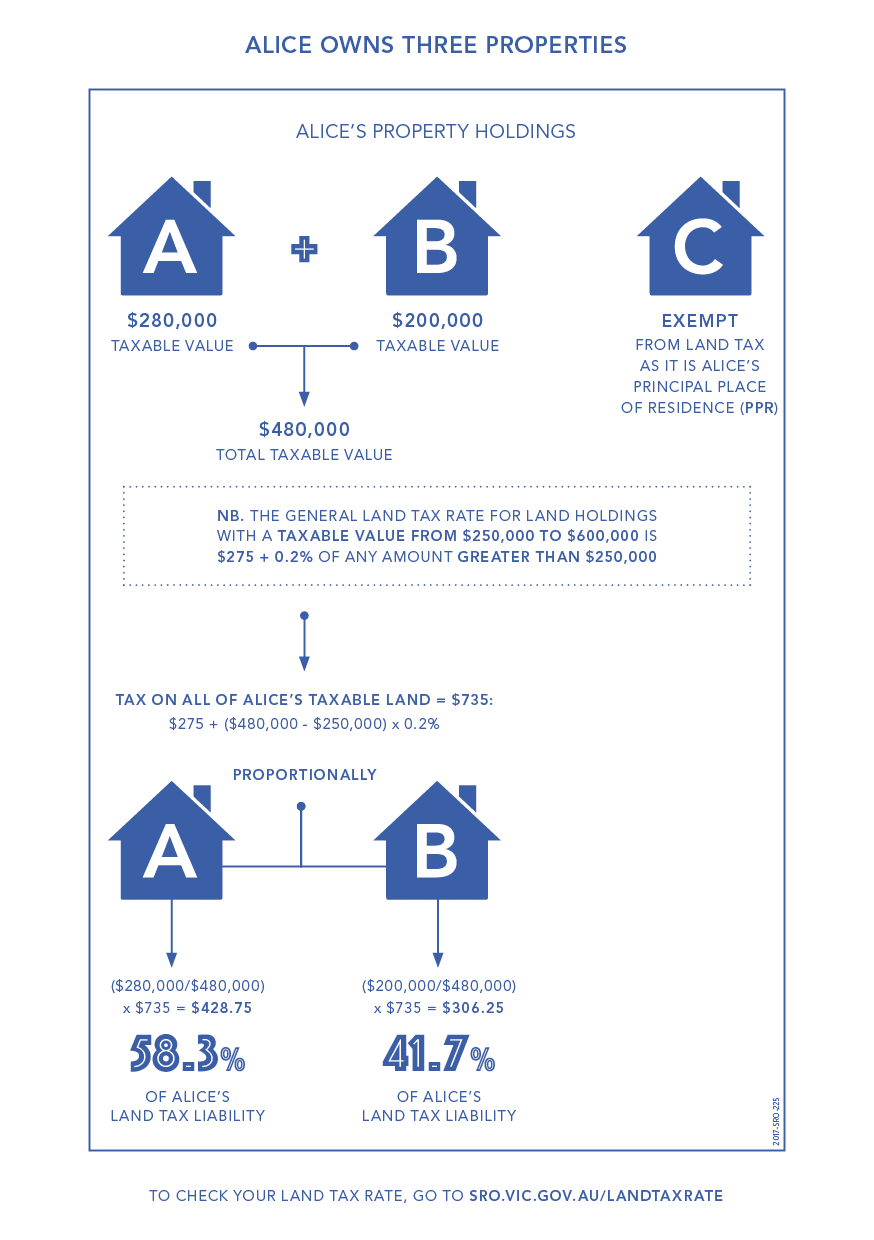### Calculating land tax

Your land tax assessment is based on the total taxable value of your land holdings as at midnight on 31 December of the preceding year. The taxable value of each property/land you own is usually the site value found on your council rate notice. Apartments have a site value and are therefore included in the total taxable value of your landholdings.

Land tax is calculated by applying the appropriate land tax rate to the total taxable value of your land holdings, excluding exempt land such as your home.

For example, at midnight on 31 December, Alice owns:

• Property A, an investment property with a taxable value of \$280,000.
• Property B, an investment property with a taxable value of \$200,000.
• Property C, her principal place of residence (PPR).

Alice’s total land tax liability for the following year is calculated by applying the appropriate land tax rate to the total taxable value of the non-exempt land she owns as at midnight on 31 December.

She is only assessed on the total taxable value of Property A and Property B, which is \$480,000 because Property C is Alice’s PPR and is therefore exempt from land tax.

The general land tax rate for land holdings valued from \$250,000 to less than \$600,000 is \$275 + 0.2% of any amount greater than \$250,000.

Therefore, Alice must pay \$735 in land tax, calculated as follows:

• \$275 + ((\$480,000 - \$250,000) x 0.2%) = \$735.

### Calculating proportional land tax

Proportional land tax is the amount of land tax applying to one particular land as a proportion of your total land tax liability. It is shown against each taxable property on the Statement of Lands page of your assessment.

Your principal place of residence and any other exempt land are not included in this calculation.

We calculate the proportional tax on land using the formula (X ÷ Y) x Z where:

• X = taxable value of a property.
• Y = total value of all taxable properties.
• Z = total tax payable.

In Alice’s case:

• Property C is exempt from land tax because it is her PPR and therefore excluded from this calculation.
• The proportional land tax payable on each of her other two properties is:
• Property A - (\$280,000 ÷ \$480,000) x \$735 = \$428.75 (i.e. 58.3% of her land tax liability).
• Property B - (\$200,000 ÷ \$480,000) x \$735 = \$306.25 (i.e. 41.7% of her land tax liability).